Total marks: --
Total time: --
INSTRUCTIONS
(1) Assume appropriate data and state your reasons
(2) Marks are given to the right of every question
(3) Draw neat diagrams wherever necessary

1 (a) Draw the F-V the F-I analogous circuit for the mechanical system shown in Fig Q1(a) with necessary equation.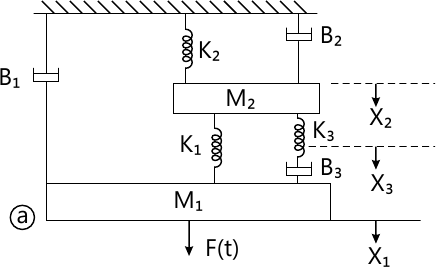12 M
1 (b) For the rotational mechanical system shown draw the torque-voltage analogous circuit for Fig Q1(b).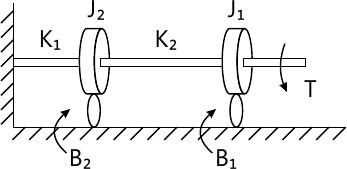8 M

2 (a) Using block diagram reduction techniques for C/R for Fig Q2(a).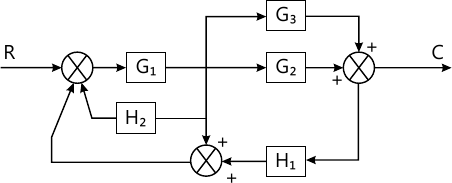5 M
2 (b) Draw the signal flow graph for the block diagram shown in Fig Q2(b) and find the TF.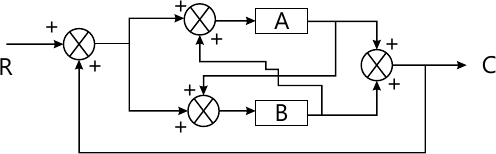8 M
2 (c) Draw the signal flow graph and find TF Fig Q2(c).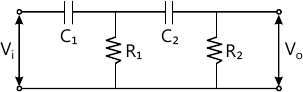7 M

3 (a) Find the error coefficients Kp, Kv and Ka for the system having $G(s)= \dfrac {10}{s^2+2s+9} \ \& \ H(s)=0.2$
6 M
3 (b) Find K1 so that ?=0.35. Find the corresponding time domain specifications for Fig Q3(b).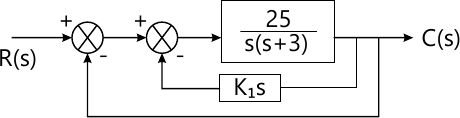6 M
3 (c) With respect to a second order system define the following by drawing neat response curve and expressions: i) Maximum overshoot (Mp); ii) Time delay (td); iii) Time constant (T); iv) Rise time (tt).
8 M

4 (a) What are the necessary and sufficient conditions for a system to be stable according to Routh-Hurwitz criterion?
4 M
4 (b) What value of K makes the following unity feedback system stable? $G(s)=\dfrac {K(s+1)^2}{s^3}$
4 M
4 (c) Find how many roots have real parts greater than -1 for the characteristics equation.
s3+7s2+25s+39=0
4 M
4 (d) How many roots of the characteristic polynomial lie in the right half of S-plane, the left half of s-plane and on jω axis. Comment on the stability of the system.
P(s)=s5+2s4+2s3+4s2+s+2
8 M

5 (a) What are the angle and magnitude conditions that a point on root locus has to satisfy?
6 M
5 (b) Sketch the root locus for the unity feedback control system whose open loop transfer function is $G(s)={1}{s(s+2)(s^2+4s+13)}$
14 M

6 (a) With respect to Nyquist criterion explain the following:
i) Encircle of a point
ii) Analytic function and its singularities.
iii) Mapping theorem of principle of argument
iv) Find the number of encirclements of point A in Fig Q6.1(a) and Q6.1(b)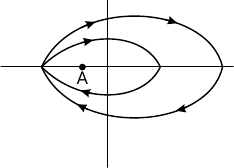8 M
6 (b) For the open loop TF of a feedback control system $G(s)H(s)=\dfrac {K(1+2s)}{s(1+s)(1+s+s^2)}.$ Sketch the complete Nyquist plot and hence find the range of K for stability using Nyquist criterion.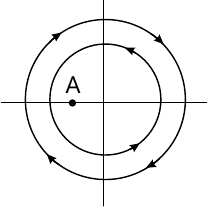12 M

7 (a) Draw the bode plot for a system having $G(s)=\dfrac {K(1+0.2s)(1+0.025s)}{s^3(1+0.01s)(1+0.05s)}.$ Comment on the stability of the system. Also find the range of K for stability.
12 M
7 (b) For the plot shown determine the TF (Fig Q7(b)).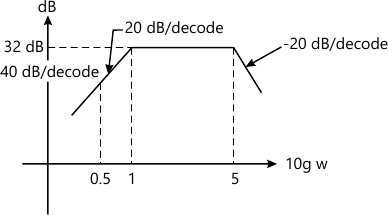8 M

8 (a) What are the advantages of state space analysis?
4 M
8 (b) A system is described by the differential equation $\dfrac {d^3y}{dt^3}+ \dfrac {3d^2y}{dt^2}+ \dfrac {17dy}{dt}+5y=10u(t)$ Where y is the output and u is the input to the system. Determine the state space representation of the system.
6 M
8 (c) Obtain the state equations for the electrical network shown in Fig. Q8(c).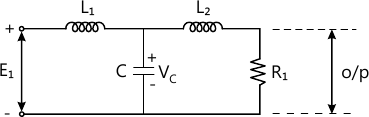10 M

More question papers from Control Systems